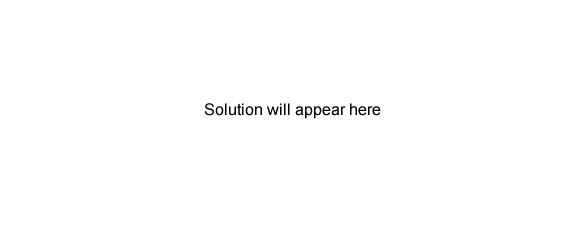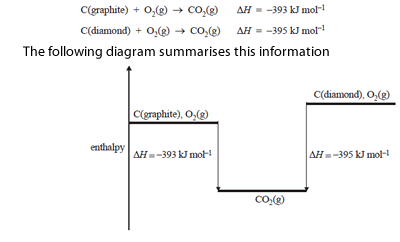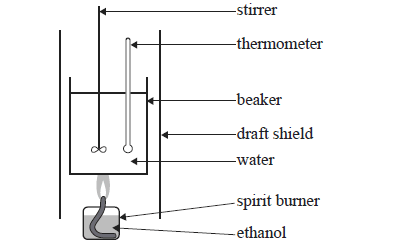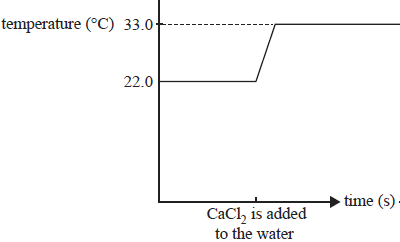Thermochemistry (2011 VCE) 1) In an endothermic reaction the a) reaction system loses energy to the surroundings. b) addition of a catalyst increases the activation energy. c) activation energy is greater than the enthalpy of reaction. d) energy required to break bonds in the reactants is less than the energy released when bonds are formed in the products. Solution. 2) Consider the following combustion reaction for graphite and diamond.From the data it can be determined that the enthalpy change, /\ H, for the conversion of graphite to diamond C(graphite) => C(diamond) is a) -2 kJ mol- b) +2kJ mol- c) -788 kJ mol- d) +788 kJ mol- Solution3) What mass of butane (M = 58.0 g mol- ) must undergo complete combustion to raise the temperature of 100.0 g of water by 1.00 oC? Assume no heat loss. Molar enthalpy of combustion for butane is 2874 kj mol- a) 8.44 g b) 6.88 g c) 0.399 g d) 8.44 X 10-3 g Solution4) A student experimentally determined the molar enthalpy of combustion of ethanol (M = 46.0 g mol-) using the equipment shown in the following simplified diagram.The student made the following experimental measurements Mass of water in beaker = 100g Amount of ethanol combusted = 0.0200 mol Temperature rise of the water = 40.0 oC The molar enthalpy of combustion of ethanol calculated from the student's results is a) -16.7 kJ mol- b) -18.2 kJ mol- c) -334 kJ mol- d) -836 kJ mol- Solution5) A solution calorimeter was calibrated by passing an electric current through the heating coil at a potential difference of 5.0 volts. This caused the in the calorimeter to increase in temperature by 9.50 oC. The calibration factor for this calorimeter was perviously determined to be 0.354 kJoC-. a) Use the calibration factor to determine the electrical charge, in coulombs, that passed through the heating coil. Solutionb) The calorimeter is then used to determine the enthalpy change for the dissolution of one mol of anhydrous calcium chloride, CaCl2 in water CaCl2 (s) => CaCl2 (aq) 6.038 g of solid anhydrous calcium chloride,CaCl2, was added to water. The mixture was stirred until all the solid had dissolved. The temperature was monitored before and after the addition of the calcium chloride. The results are shown below.b) Is this reaction exothermic or endothermic? Explain. Solution c) Use the calibration factor to calculate the enthalpy change for the dissolution of 1.00 mol of CaCl2(s). The molar mass of CaCl2 = 111.1 g/mol Solution